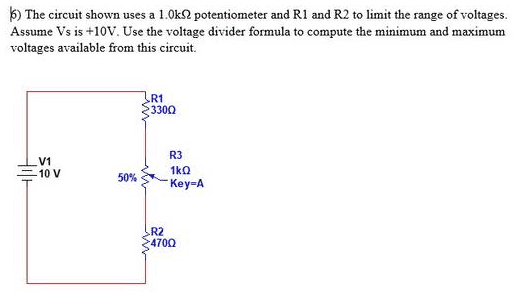# I can't figure out what resistance values to use....

• Megatronlol
In summary, the conversation focused on a problem involving a potentiometer and the confusion on how to compute voltages in a circuit. The individual had mistakenly assumed that the potentiometer was wired as a variable resistor and had used incorrect values in their calculations. It was clarified that the potentiometer value remains constant and its position changes the fraction of resistance above and below the wiper. The correct approach is to model the circuit as two resistors and use the potential divider equation to calculate the voltage output.

#### Megatronlol

Thread moved from the technical forums, so no Homework Template is shownI started an electronics program recently and I am a month into it so I am quite new at this. I have a problem involving a potentiometer and I don't understand how to compute the voltages because I don't know what I am supposed to use for my resistance values.

I know that in series the total resistance is the sum of the individual resistors. I assume that since R1 = 330Ω and R2 = 470Ω that I am taking the pot from 1Ω to 1000Ω and using a minimum value of 801Ω and a maximum of 1800Ω and using my voltage divider formula but that answers that I get don't match the measured values from the lab. In the lab I measured a maximum voltage of 8.13V and a minimum voltage of 2.65V. Can anyone offer any insight as to what resistor values I would be using for my voltage divider formula and why those values? Thanks.

https://imgur.com/a/BCk2QVg here is a link to the problem and circuit#### Attachments

Last edited by a moderator:
When the pot is at the top of its travel, the upper resistance of the divider is 330R and the lower 1470R So the output voltage will be 10 x Lower/(Upper + Lower).
When the pot is at the bottom of its travel, the upper resistance is 1330R and the lower resistance is 470R, and the output voltage can be calculated in the same way.

You need to show us your calculations before we are allowed to help.

anorlunda said:
You need to show us your calculations before we are allowed to help.

Normally I would agree but they have shown they don't understand how the pot is working in this circuit with the following, so their calculations are going to be totally wrong.

Megatronlol said:
I know that in series the total resistance is the sum of the individual resistors. I assume that since R1 = 330Ω and R2 = 470Ω that I am taking the pot from 1Ω to 1000Ω and using a minimum value of 801Ω and a maximum of 1800Ω and using my voltage divider formula

That's wrong. The pot is NOT wired up as a variable resistor.

The value of the pot doesn't actually change in this circuit. It's value is a constant 1000R but when you move the wiper the fraction "below" the wiper (which is the output node) compared to "above" it changes. So for example with the wiper in the middle the pot (alone) can be modeled as two 500R resistors.

The whole circuit could then be modeled as (a different) two resistors which can be used in the potential divider equation.

330+500 = 830R
and
470+500 = 970R

Obviously your numbers would be different because they don't ask for the voltage with the wiper in the middle.